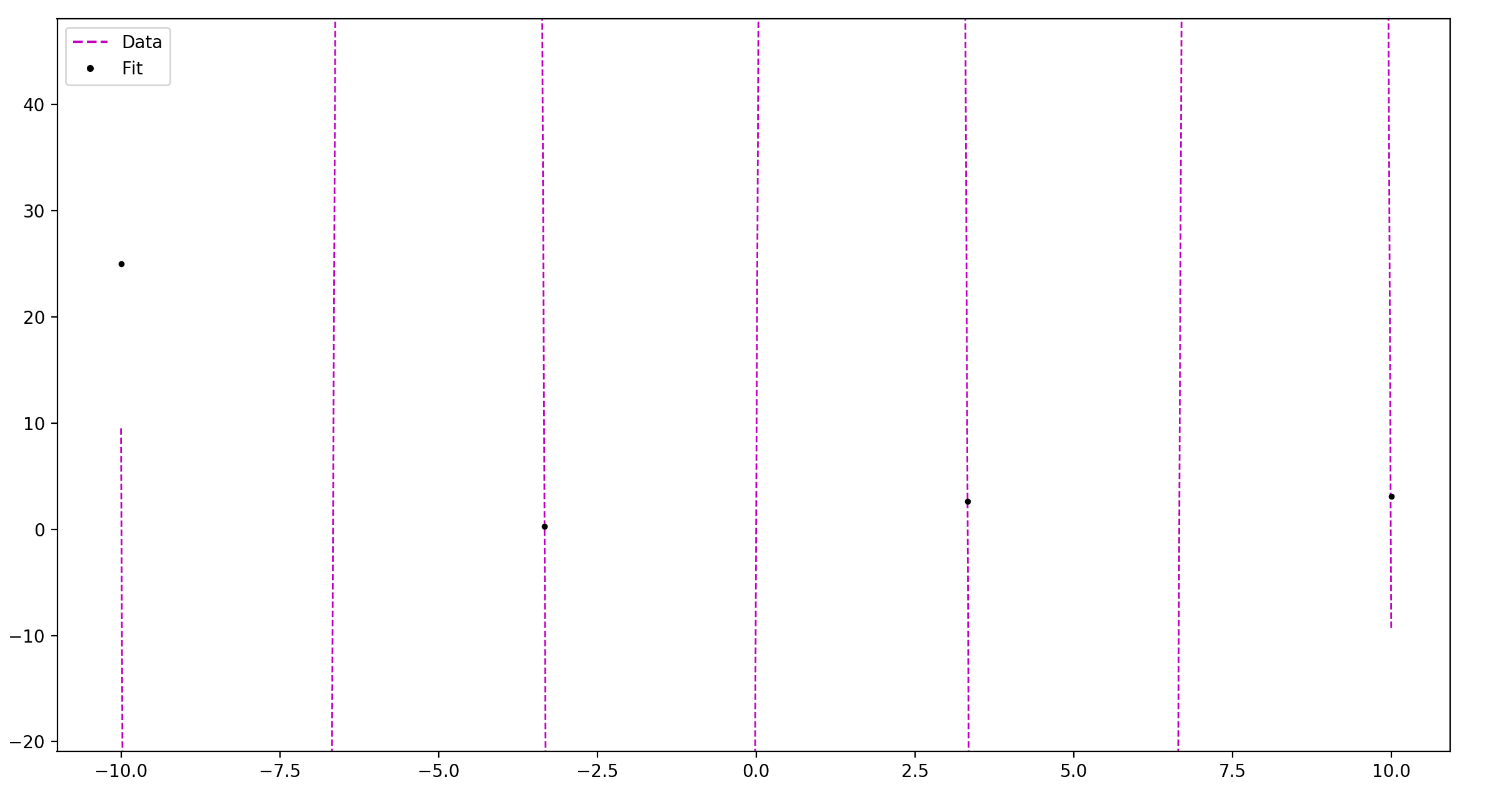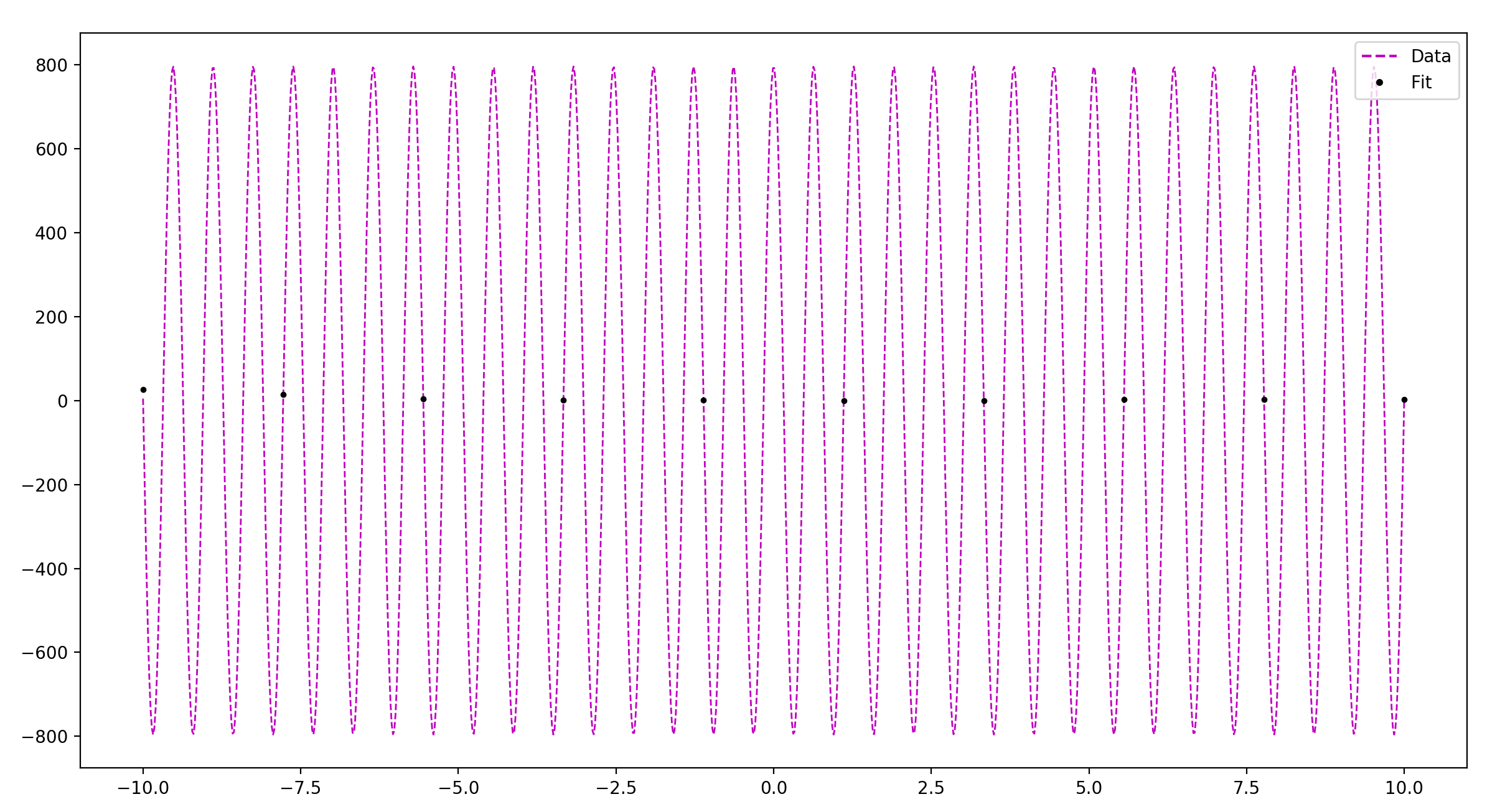# Why “number of parameters” isn’t good enough

A friend of mine recently pointed out a curious fact. Any set of two-dimensional data whatsoever can be perfectly fit by a simple two-parameter sinusoidal model.

y(x) = A sin(Bx)

Sound wrong? Check it out:Zoomed out:N = 10 pointsAs you see, as the number of data points goes up, all you need to do to accommodate this is increase the frequency in your sine function, and adjust the amplitude as necessary. Ultimately, you can fit any data set with a ridiculously quickly oscillating and large-amplitude sine function.

Now, most model selection methods explicitly rely on the parameter count to estimate the potential of a model to overfit. For example, if k is the number of parameters in a model, and L is the log likelihood of the data given the model, we have:

AIC = L – k
BIC = L – k/2・log(N)

This little example represents a fantastic failure of parameter count to successfully do the job AIC and BIC ask of it. Evidently parameter count is too blunt an instrument to do the job we require of it, and we need something with more nuance.

One more example.

For any set of data, if you can perfectly fit a curve to each data point, and if your measurement error σ is an adjustable parameter, then you can take the measurement error to zero to have a fit with infinite accuracy. Now when we evaluate, you find it running off to infinity! Thus our ‘fit to data’ term L goes to infinity, while the model complexity penalty stays a small finite number.

Once again, we see the same lack of nuance dragging us into trouble. The number of parameters might do well at estimating overfitting potential for some types of well-behaved parameters, but it clearly doesn’t do the job universally. What we want is some measure that is sensitive to the potential for some parameters to capture “more” of the space of all possible distributions than others.

And lo and behold, we have such a measure! This is the purpose of information geometry and the volume of a model in the space formed by the Fisher information metric as the penalty for overfitting potential. You can learn more about it in a post I wrote here.